Previous: 6.2.4 Probabilistic Structure Up: 6.2 Quantum Mechanics in Next: 6.2.6 A Feasible Phase

## 6.2.5 A Caveat on Marginal Distributions

If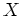andare random variables and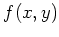is their joint probability distribution, the marginal distribution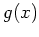ofis given by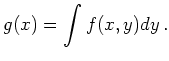(6.54)

It can be interpreted as a probability density of the single variable x. The random variablesandare independent if and only if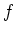can be factorized by the marginal distributions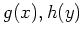as(6.55)

The Wigner distributionhas the properties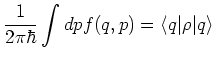(6.56)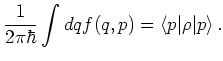(6.57)

For an observable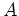with corresponding symbolwe have for its expectation value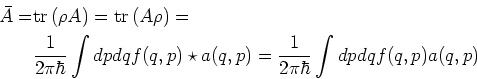(6.58)

where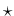denotes the Moyal star product.

Classically the definition of the local (marginal) expectation values of observables is not ambiguous due to the commutativity of all observables. However, quantum mechanically to each way of calculating the expectation value in 6.59 corresponds a definition for the local expectation value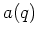and in general these definitions give different results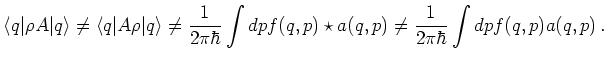(6.59)

In general the operator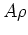is not selfadjoint, so the definitions using Dirac brackets above are different. We can rephrase this by using the explicit expression for the density operator for a pure state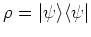. For a single wave function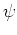we get two definitions for the local expectation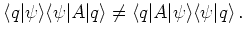(6.60)

A good way to define the local expectation is to symmetrize the definitions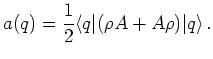(6.61)

This definition has the property that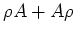is again a selfadjoint operator, hence the local expectation is real. This is the way in which the current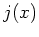is conventionally defined.

A special case which is not treated in this way is the definition of local expectation for an energy-like operator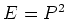where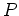is selfadjoint. Then we can define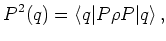(6.62)

which is a positive quantity. We use this form to define a positive phase space distribution function in Section 6.2.6.

However, in the Wigner formalism one usually defines energy as the second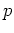-moment in the form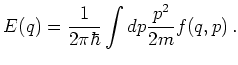(6.63)

With this definition for the local expectation value the local energy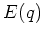can become negative, as was observed in Wigner function simulations.

To calculate the Wigner transformation of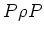we use a suitable definition for the star product. We get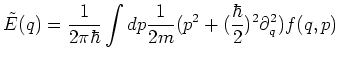(6.64)

which is seen to differ from the second order moment definition 6.64.

The question of marginalization is also discussed in [Wlo99].